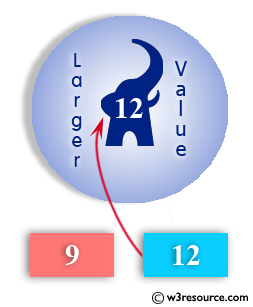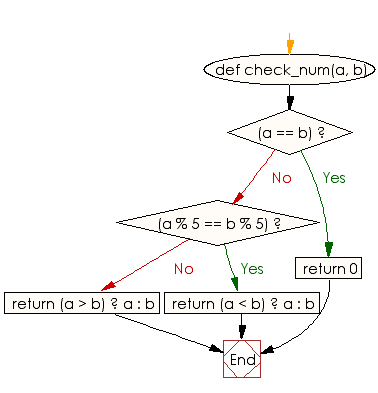﻿ Ruby Basic exercises: Check two given integers and return the larger value - w3resource# Ruby Basic Exercises: Check two given integers and return the larger value

## Ruby Basic: Exercise-50 with Solution

Write a Ruby program to check two given integers and return the larger value. However if the two values have the same remainder when divided by 5 then return the smaller value and if the two values are the same, return 0.Ruby Code:

``````def check_num(a, b)
if(a == b)
return 0
end
if(a % 5 == b % 5)
return (a < b) ? a : b
end
return (a > b) ? a : b
end

print check_num(9, 12),"\n"
print check_num(110, 200),"\n"
print check_num(10, 10)
``````

Output:

```12
110
0
```

Flowchart:Ruby Code Editor: